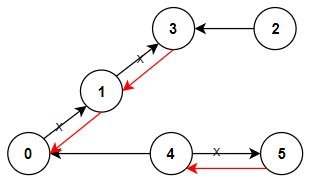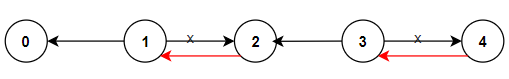# 1466. Reorder Routes to Make All Paths Lead to the City Zero

Medium
There are `n` cities numbered from `0` to `n - 1` and `n - 1` roads such that there is only one way to travel between two different cities (this network form a tree). Last year, The ministry of transport decided to orient the roads in one direction because they are too narrow.
Roads are represented by `connections` where `connections[i] = [ai, bi]` represents a road from city `ai` to city `bi`.
This year, there will be a big event in the capital (city `0`), and many people want to travel to this city.
Your task consists of reorienting some roads such that each city can visit the city `0`. Return the minimum number of edges changed.
It's guaranteed that each city can reach city `0` after reorder.
Example 1:Input: n = 6, connections = [[0,1],[1,3],[2,3],[4,0],[4,5]]
Output: 3
Explanation: Change the direction of edges show in red such that each node can reach the node 0 (capital).
Example 2:Input: n = 5, connections = [[1,0],[1,2],[3,2],[3,4]]
Output: 2
Explanation: Change the direction of edges show in red such that each node can reach the node 0 (capital).
Example 3:
Input: n = 3, connections = [[1,0],[2,0]]
Output: 0
Constraints:
• `2 <= n <= 5 * 10^4`
• `connections.length == n - 1`
• `connections[i].length == 2`
• `0 <= ai, bi <= n - 1`
• `ai != bi`

### 解題

type Path struct {
n int
dist int
}
func minReorder(n int, connections [][]int) int {
graph := make([][]Path, n)
for _, conn := range connections {
graph[conn] = append(graph[conn], Path{ n: conn, dist: 1})
graph[conn] = append(graph[conn], Path{ n: conn, dist: 0})
}
queue := make([]Path, 0)
queue = append(queue, Path{ n: 0, dist: 0})
visited := make([]bool, n)
ans := 0
for len(queue) != 0 {
top := queue
queue = queue[1:]
visited[top.n] = true
ans += top.dist
for _, p := range graph[top.n] {
if visited[p.n] { continue }
queue = append(queue, p)
}
}
return ans
}